# Euler–Mascheroni constant facts for kids

Kids Encyclopedia Facts

The Euler-Mascheroni constant is a number that appears in analysis and number theory. It first appeared in the work of Swiss mathematician Leonhard Euler in the early 18th century. It is usually represented with the Greek letter,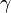$\gamma$, although Euler used the letters C and O instead. It is not known yet whether the number is irrational (which would mean that it cannot be written as a fraction with an integer numerator and denominator) and/or transcendental (which would mean that it is not the solution of a polynomial with integer coefficients). The numerical value of$\gamma$ is about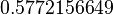$0.5772156649$. Italian mathematician Lorenzo Mascheroni also worked with the number, and tried, unsuccessfully, to approximate the number to 32 decimal places, making mistakes on five digits.

It is significant because it links the divergent harmonic series with the natural logarithm. It is given by the limiting difference between the natural logarithm and the harmonic series :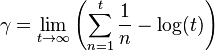$\gamma = \lim_{t \to \infty} \left(\sum_{n=1}^{t} \frac{1}{n} - \log(t)\right)$

It can also be written as an improper integral involving the floor function, which gives the greatest integer less than or equal to a given number.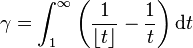$\gamma = \int_{1}^{\infty} \left(\frac{1}{\lfloor t \rfloor} - \frac{1}{t}\right) \mathrm{d}t$

The gamma constant is closely linked to the Gamma function , specifically its logarithmic derivative, the digamma function, which is defined as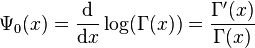$\mathrm{\Psi}_0(x) = \frac{\mathrm{d}}{\mathrm{d}x} \log(\Gamma(x)) = \frac{\Gamma'(x)}{\Gamma(x)}$

For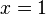$x=1$, this gives us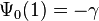$\mathrm{\Psi}_0(1) = -\gamma$

Using properties of the digamma function,$\gamma$ can also be written as a limit.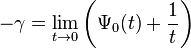$-\gamma = \lim_{t \to 0} \left(\mathrm{\Psi}_0(t) + \frac{1}{t}\right)$Euler–Mascheroni constant Facts for Kids. Kiddle Encyclopedia.